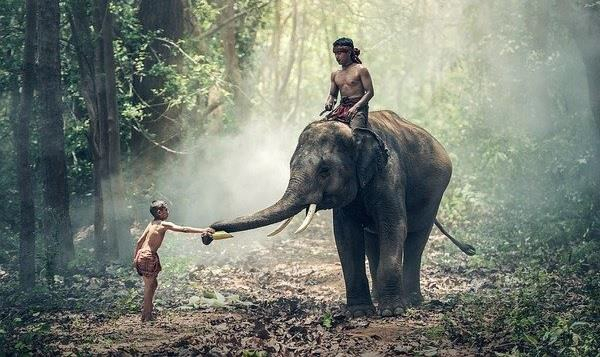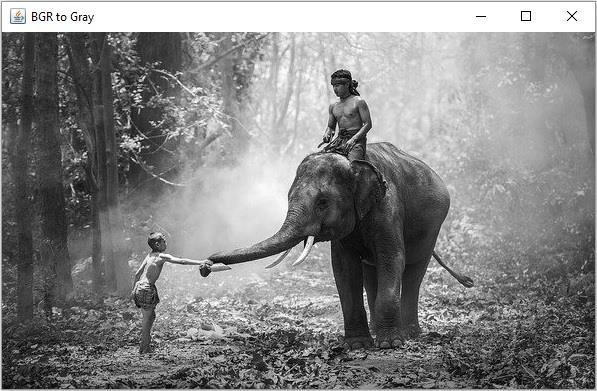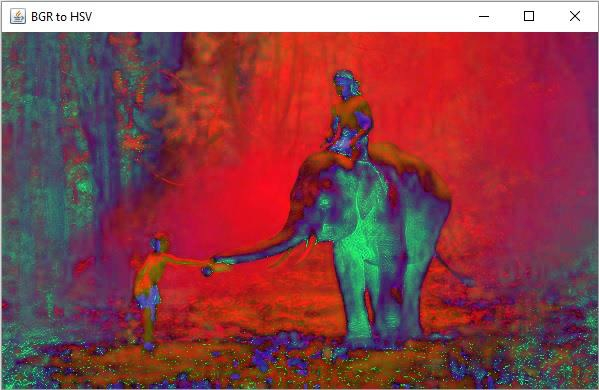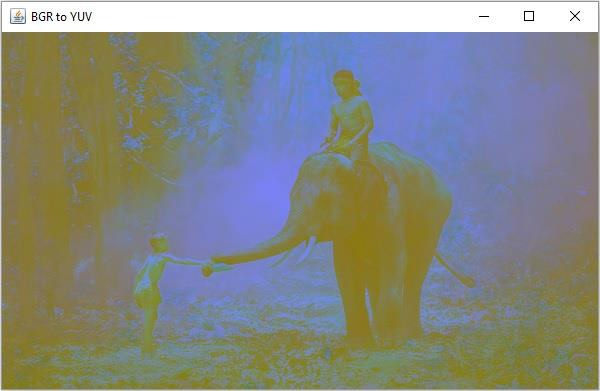# How to change the color spaces of an image using Java OpenCV library?

Using color space protocol you can represent the colors in an image. There are several color spaces available in OpenCV some of them are −

• BGR − RGB is the most widely used color space in this, each pixel is actually formed by three different colors (intensity) values: red, blue and green, it is the default color space in OpenCV but it is stored as BGR.

• HSV − In HSV color space the different colors are formed by changing the hue, saturation, and brightness.

• CMK − This is a subtractive color space, in this the different colors are formed by subtracting the Cyan, Magenta and Yellow values, starting from white.

• Y’UV − Y’UV defines a color space in terms of one luma (Y’) and two chrominance (UV) components. The Y’UV color model is used in the following composite color video standards.

You can convert the representation of an image from one color space to another using the cvtColor() method of the org.opencv.imgproc.Imgproc class. This method accepts a source image, destination image and the code representing the color of the destination image.

To change the color space from BGR to HSV you need to pass COLOR_BGR2HSV as the color code value. Similarly to change the color space from BGR to YUV you need to pass COLOR_BGR2YUV as the color code.

## Example

import org.opencv.core.Core;
import org.opencv.core.Mat;
import org.opencv.highgui.HighGui;
import org.opencv.imgcodecs.Imgcodecs;
import org.opencv.imgproc.Imgproc;
public class ChangingColorSpaces {
public static void main(String args[]) throws Exception {
//Creating the empty destination matrix
Mat dst = new Mat();
//Converting From BGR to Gray
Imgproc.cvtColor(src, dst, Imgproc.COLOR_BGR2GRAY);
HighGui.imshow("BGR to Gray", dst);
dst = new Mat();
//Converting From BGR to HSV
Imgproc.cvtColor(src, dst, Imgproc.COLOR_BGR2HSV);
HighGui.imshow("BGR to HSV", dst);
dst = new Mat();
//Converting From BGR to HSV
Imgproc.cvtColor(src, dst, Imgproc.COLOR_RGB2YUV);
HighGui.imshow("BGR to YUV", dst);
HighGui.waitKey();
}
}

## Input Image## Output

On executing, the above program generates the following windows −

BGR to Gray −BGR to HSV −BGR to YUV −